# ML Aggarwal Class 10 Solutions for ICSE Maths Chapter 9 Arithmetic and Geometric Progressions Ex 9.4

## ML Aggarwal Class 10 Solutions for ICSE Maths Chapter 9 Arithmetic and Geometric Progressions Ex 9.4

ML Aggarwal Class 10 Solutions for ICSE Maths Chapter 9 Arithmetic and Geometric Progressions Ex 9.4

Question 1.
Can 0 be a term of a geometric progression?
Solution:
No, 0 is not a term of geometric progression.

Question 2.
(i) Find the next term of the list of numbers $$\frac { 1 }{ 6 } ,\frac { 1 }{ 3 } ,\frac { 2 }{ 3 } ,…$$
(ii) Find the next term of the list of numbers $$\frac { 3 }{ 16 } ,-\frac { 3 }{ 8 } ,\frac { 3 }{ 4 } ,-\frac { 3 }{ 2 } ,…$$
(iii) Find the 15th term of the series $$\sqrt { 3 } +\frac { 1 }{ \sqrt { 3 } } +\frac { 1 }{ 3\sqrt { 3 } } +…$$
(iv) Find the nth term of the list of numbers $$\frac { 1 }{ \sqrt { 2 } } ,-2,4\sqrt { 2 } ,-16,…$$
(v) Find the 10th and nth terms of the list of numbers 5, 25, 125, …
(vi) Find the 6th and the nth terms of the list of numbers $$\frac { 3 }{ 2 } ,\frac { 3 }{ 4 } ,\frac { 3 }{ 8 } ,…$$
(vii) Find the 6th term from the end of the list of numbers 3, – 6, 12, – 24, …, 12288.
Solution: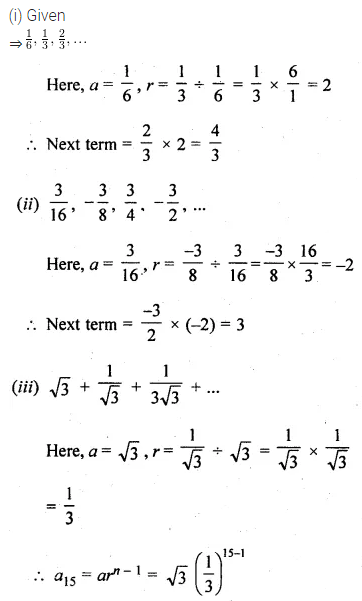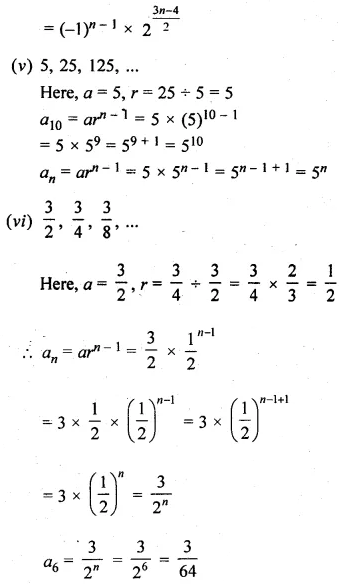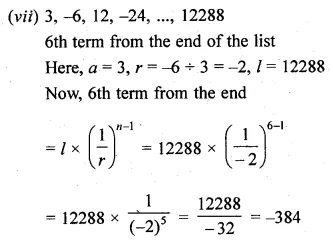Question 3.
Which term of the G.P.
(i) 2, 2√2, 4, … is 128?
(ii) $$1,\frac { 1 }{ 3 } ,\frac { 1 }{ 9 } ,…is\quad \frac { 1 }{ 243 } ?$$
(iii) $$\frac { 1 }{ 3 } ,\frac { 1 }{ 9 } ,\frac { 1 }{ 27 } ,…is\quad \frac { 1 }{ 19683 } ?$$
Solution: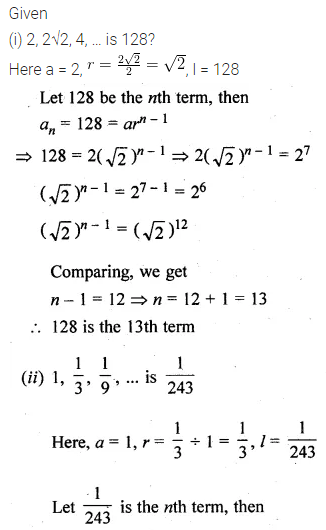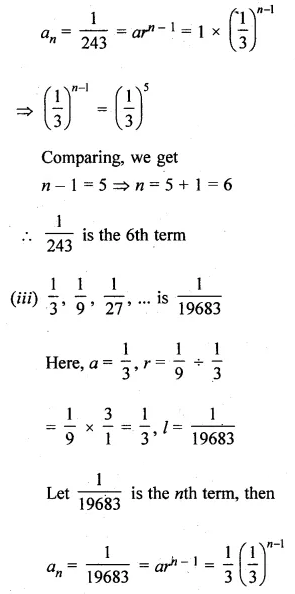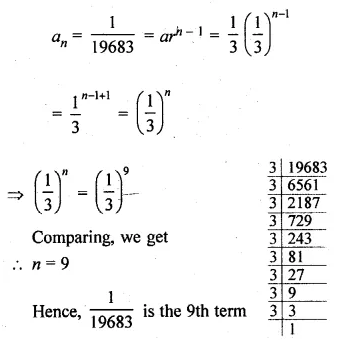Question 4.
Which term of the G.P. 3, -3√3, 9, -9√3, … is 729?
Solution: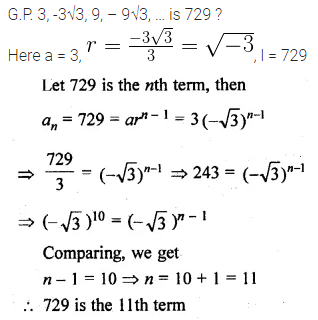Question 5.
Determine the 12th term of a G.P. whose 8th term is 192 and the common ratio is 2.
Solution:Question 6.
In a GP., the third term is 24 and 6th term is 192. Find the 10th term
Solution: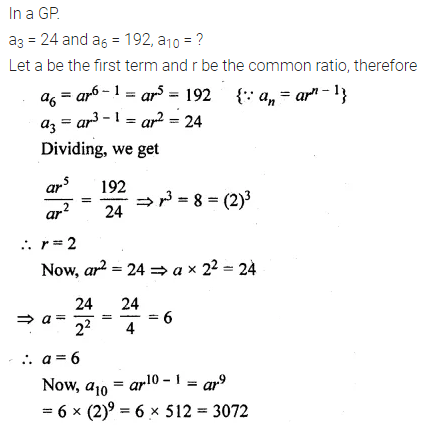Question 7.
Find the number of terms of a G.P. whose first term is $$\\ \frac { 3 }{ 4 }$$, common ratio is 2 and the last term is 384.
Solution:Question 8.
Find the value of x such that
(i) $$-\frac { 2 }{ 7 } ,x,-\frac { 7 }{ 2 }$$ are three consecutive terms of a G.P.
(ii) x + 9, x – 6 and 4 are three consecutive terms of a G.P.
(iii) x, x + 3, x + 9 are first three terms of a G.P. Find the value of x.
Solution:Question 9.
If the fourth, seventh and tenth terms of a G.P. are x, y, z respectively, prove that x, y, z are in G.P.
Solution: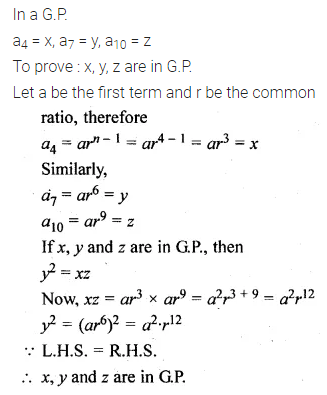Question 10.
The 5th, 8th and 11th terms of a G.P. are p, q, and s respectively. Show that q² = ps.
Solution: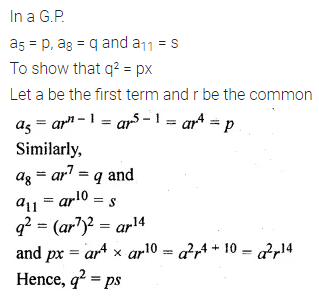Question 11.
If a, b, c are in G.P., then show that a², b², c² are also in G.P.
Solution: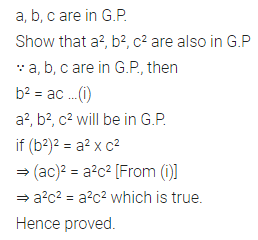Question 12.
If a, b, c are in A.P., then show that 3a, 3b, 3c are in G.P.
Solution: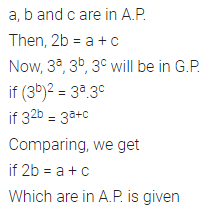Question 13.
If a, b, c are in A.P., then show that 10ax + 10, 10bx + 10, 10cx + 10, x ≠ 0, are in G.P.
Solution: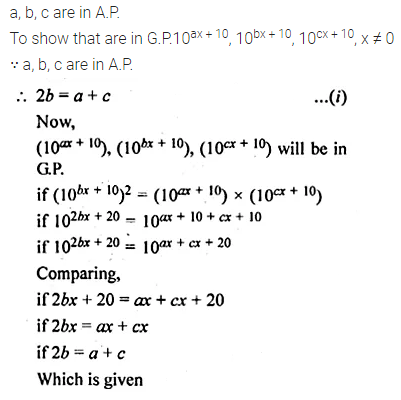Question 14.
If a, a2+ 2 and a3 + 10 are in G.P., then find the values(s) of a.
Solution: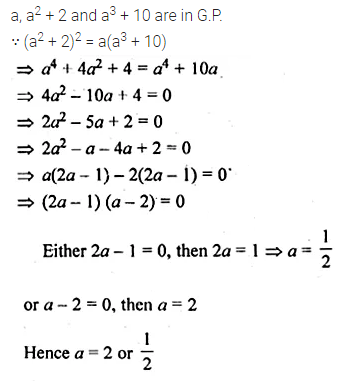Question 15.
If k, 2k + 2, 3k + 3, … are in G.P., then find the common ratio of the G.P.
Solution: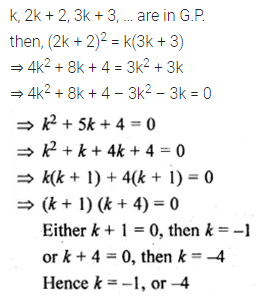Question 16.
The first and the second terms of a GP. are x-4 and xm. If its 8th term is x52, then find the value of m.
Solution: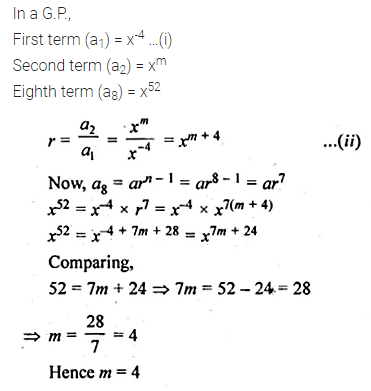Question 17.
Find the geometric progression whose 4th term is 54 and the 7th Term is 1458.
Solution: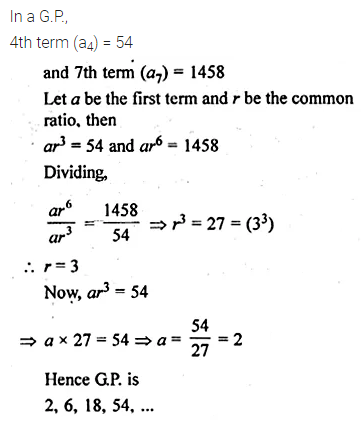Question 18.
The fourth term of a GP. is the square of its second term and the first term is -3. Determine its seventh term.
Solution: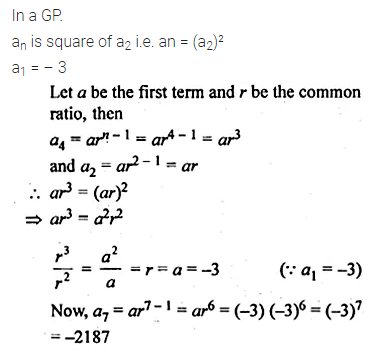Question 19.
The sum of first three terms of a G.P. is $$\\ \frac { 39 }{ 10 }$$ and their product is 1. Find the common ratio and the terms.
Solution: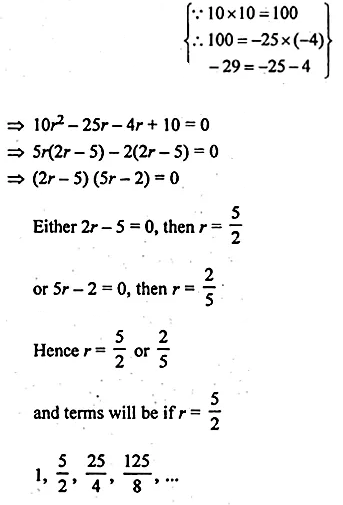Question 20.
Three numbers are in A.P. and their sum is 15. If 1, 4 and 19 are added to these numbers respectively, the resulting numbers are in G.P. Find the numbers.
Solution: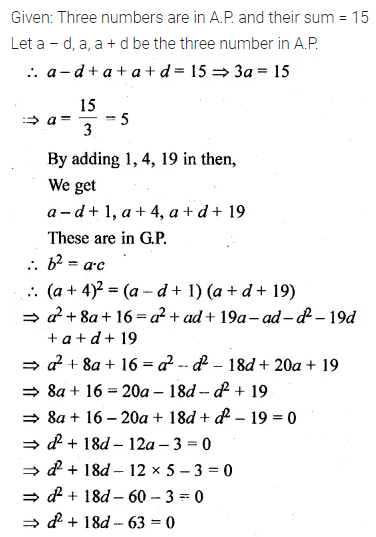Question 21.
Three numbers form an increasing G.P. If the middle term is doubled, then the new numbers are in A.P. Find the common ratio of the G.P.
Solution: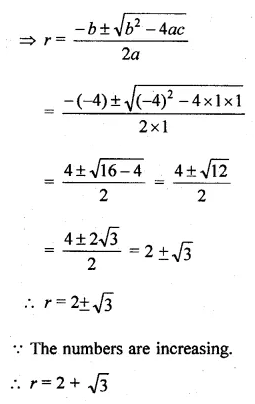Question 22.
Three numbers whose sum is 70 are in GP. If each of the extremes is multiplied by 4 and the mean by 5, the numbers will be in A.P. Find the numbers.
Solution: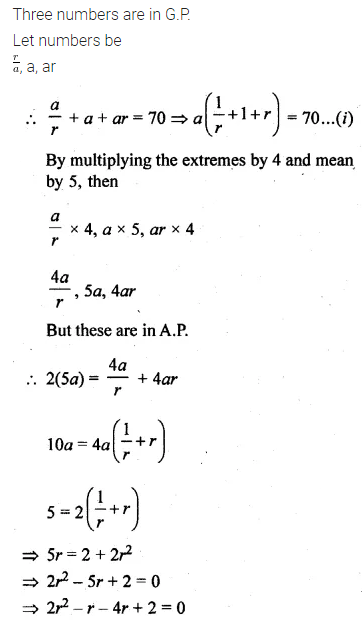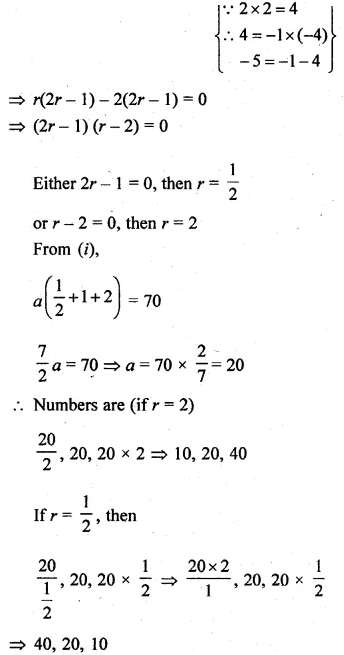Question 23.
There are four numbers such that the first three of them form an A.P. and the last three form a GP. The sum of the first and third number is 2 and that of the second and fourth is 26. What are these numbers?
Solution:Question 24.
(i) If a, b, c are in A.P. as well in G.P., prove that a = b = c.
(ii) If a, b, c are in A.P as well as in G.P., then find the value of ab-c + bc-a + ca-b
Solution:Question 25.
The terms of a G.P. with first term a and common ratio r are squared. Prove that resulting numbers form a G.P. Find its first term, common ratio, and the nth term.
Solution: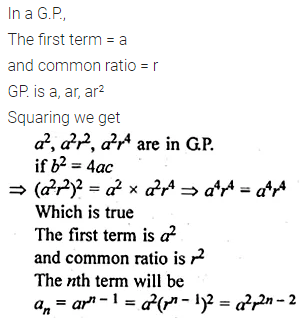Question 26.
Show that the products of the corresponding terms of two G.P.’s a, ar, ar², …, arn-1 and A, AR, AR2, …, ARn-1 form a G.P. and find the common ratio.
Solution:Question 27.
(i) If a, b, c are in G.P. show that $$\frac { 1 }{ a } ,\frac { 1 }{ b } ,\frac { 1 }{ c }$$ are also in G.P.
(ii) If K is any positive real number and Ka, Kb Kc is three consecutive terms of a G.P., prove that a, b, c are three consecutive terms of an A.P.
(iii) If p, q, r are in A.P., show that pth, qth and rth terms of any G.P. are themselves in GP.
Solution: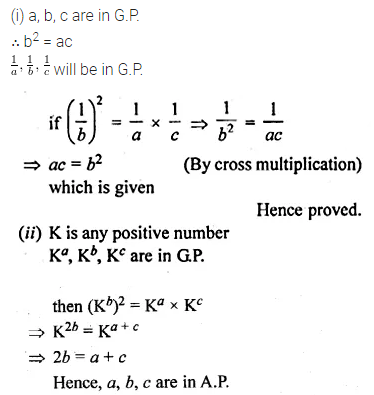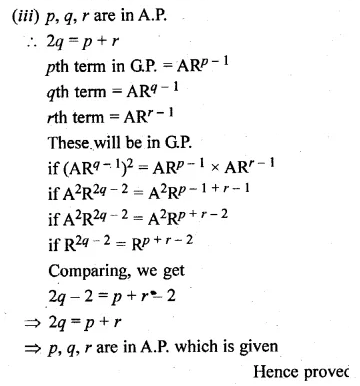Question 28.
If a, b, c are in GP., prove that the following are also in G.P.
(i) a3, b3, c3
(ii) a2 + b2, ab + bc, b2 + c2.
Solution:Question 29.
If a, b, c, d are in G.P., show that
(i) a2 + b2, b2 + c2, c2 + d2 are in G.P.
(ii) (b – c)2 + (c – a)2 + (d – b)2 = (a – d)2.
Solution: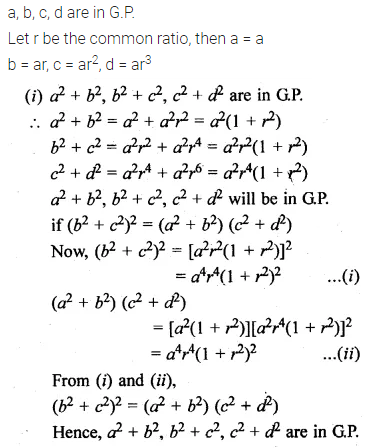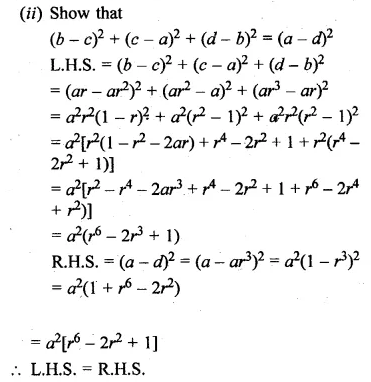Question 30.
The number of bacteria in a certain culture doubles every hour. If there were 30 bacteria present in the culture originally, how many bacteria will be present at the end of the 2nd hour, 4th hour and nth hour?
Solution:Question 31.
The length of the sides of a triangle form a G.P. If the perimeter of the triangle is 37 cm and the shortest side is of length 9 cm, find the lengths of the other two sides.
Solution: